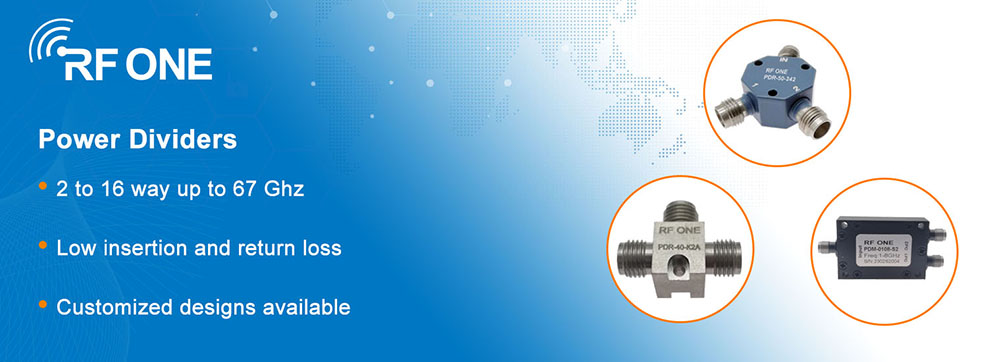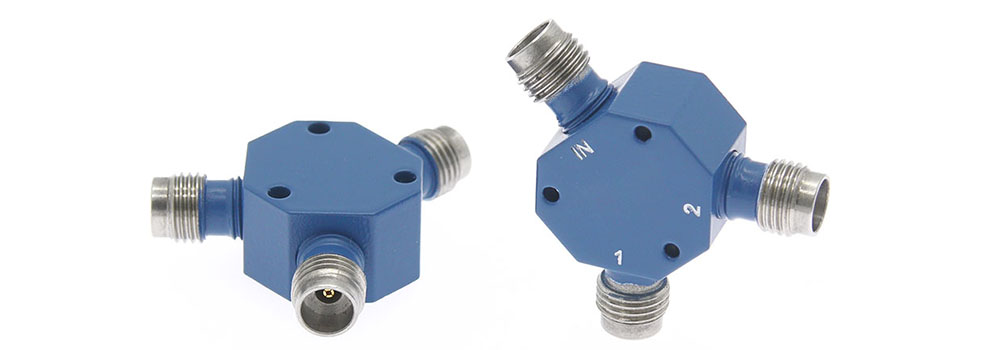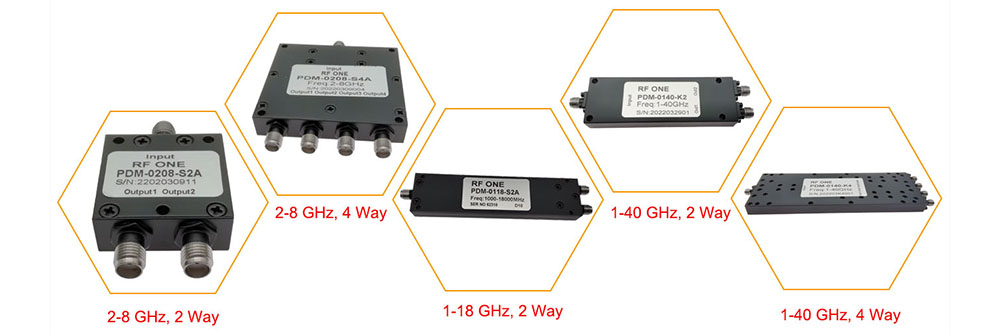18 July 2023

# Choosing the Right Power Divider for Your RF System

Introduction
Power dividers, also known as power splitters, are essential components in RF (Radio Frequency) and microwave systems. They play a critical role in splitting the input power into multiple output signals with precise power distribution ratios. The choice of a power divider type depends on various factors such as application requirements, frequency range, and power handling etc. In this article, we will explore the different types of power dividers commonly used in RF systems, discussing their principles of operation, advantages, and typical applications.

Types of Power Dividers
RF ONE offers both resistive power dividers and Wilkinson power dividers. Both types can be used to fan-in or fan-out RF signals. We offer a wide variety of configurations, including 2-way through 16-way power dividers, to accommodate various signal distribution requirements. With connector options such as 1.85mm, 2.4mm, 2.92mm, BNC, N, SMA, TNC, and 7/16 DIN, you can seamlessly integrate our power dividers into your existing system setup.RF ONE offer a wide selection of power dividers

Resistive Power Divider

A resistive power divider, is a type of power divider that utilizes resistive elements to split the input power into multiple output signals. It consists of a network of resistors connected in a specific configuration to achieve equal power division among the output ports. They are available with two or more outputs and operate from DC to several Gigahertz or more. They are low cost, small size dividers that are frequently used in low power applications where their losses can be afforded and where their low cost and broadband properties are attractive.RF ONE 2.4mm resistive power divider
Wilkinson Power Divider

A Wilkinson power divider is a type of power divider that employs quarter-wave transmission line sections and impedance transformations to achieve power splitting. Wilkinson power dividers are commonly used in RF and microwave systems where equal power division and good isolation are required.RF ONE Wilkinson Power Divider

The main differences between resistive power dividers and Wilkinson power dividers are that Wilkinson power dividers have 3 dB lower loss and possess the advantage of isolation between output ports. Practically speaking, Wilkinson power dividers are limited in their low frequency range (fLOW) to a few hundred MHz while resistive power dividers reach to DC.

How to Choose a Power Divider
When selecting a power divider for your specific application, there are several important factors to consider. These factors will impact the performance, durability, and cost-effectiveness of the device. Let’s take a look at some of the most important factors that should be considered when choosing a power divider.

Frequency Range

Consider the frequency range of your RF system when selecting a power divider. Different dividers are designed to operate within specific frequency ranges. Ensure that the power divider you choose can handle the frequencies required for your application. It is crucial to match the divider's frequency range with your system's frequency band to ensure optimal performance and signal integrity.

VSWR/Return Loss

The voltage standing wave ratio (VSWR) and return loss are metrics that indicate how well the RF network is matched to the load and source impedance. It is important to ensure a good 50-ohm match across all frequencies to achieve the best performance and minimize reflections within the system. It is essential to select a power divider with excellent return loss performance for optimal performance.

Insertion Loss

Insertion loss refers to the power loss that occurs when the signal passes through the power divider. It is crucial to minimize insertion loss to maintain the desired power distribution. Evaluate the insertion loss specifications provided by the manufacturer and choose a power divider with the lowest possible value for your specific application. Lower insertion loss ensures efficient power distribution and reduces signal degradation.

Isolation

Isolation is the degree to which the output ports of a power divider are isolated from each other. It measures how effectively the power divider prevents interference or crosstalk between the output signals. High isolation is essential to ensure that the output signals remain independent and do not interfere with each other. Consider the isolation specifications of the power divider and select one that offers adequate isolation for your application requirements.

Amplitude Balance

Amplitude balance is a measure of how evenly the power is split between the two arms of the device. The amplitude balance is typically less than 0.3 dB for RF ONE Wilkinson power dividers.

Phase Balance

Phase balance is a measure of the differential phase shift between the two output arms of the power divider. The Phase balance is typically less than 3 degree for RF ONE Wilkinson power dividers. Evaluate the phase balance specifications based on your application's requirements.

Power Handling Capability

Power handling capability determines the maximum power level that a power divider can handle without degrading its performance or causing damage. It is crucial to select a power divider that can handle the power levels required for your specific application. Consider factors such as peak power, average power, and power distribution requirements to ensure that the selected power divider can handle the power levels effectively.

Conclusion
Power dividers play a vital role in RF systems, enabling accurate signal distribution and power division. Understanding the fundamentals of power dividers, their types, applications, and considerations for selection is crucial for optimizing system performance. By considering factors such as frequency range, power handling capability, insertion loss, isolation, and impedance matching, you can choose the right power divider for your specific needs. If you have any needs for power dividers, please visit www.rfone.cn or contact us via sales@rfone.cn.
CATEGORIES